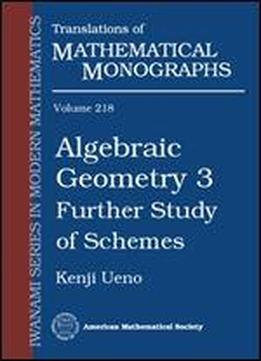# Algebraic Geometry 3 Further Study Of Schemes (translations Of Mathematical Monographs) by Kenji Ueno / 2003 / English / DjVu

Algebraic geometry plays an important role in several branches of science and technology. This is the last of three volumes by Kenji Ueno algebraic geometry. This, in together with Algebraic Geometry 1 and Algebraic Geometry 2, makes an excellent textbook for a course in algebraic geometry. In this volume, the author goes beyond introductory notions and presents the theory of schemes and sheaves with the goal of studying the properties necessary for the full development of modern algebraic geometry. The main topics discussed in the book include dimension theory, flat and proper morphisms, regular schemes, smooth morphisms, completion, and Zariski's main theorem. Ueno also presents the theory of algebraic curves and their Jacobians and the relation between algebraic and analytic geometry, including Kodaira's Vanishing Theorem.

views: 359